• cout特征向量为:\n"; for(i=0;i;i++) { cout("; for(j=0;j;j++) { cout(10)[j][i]; } cout)T"; cout; } }   图片说明]...c 矩阵
• 目录 前景提要 ...可以看一下前一篇【Python】当你需要计算1,000,000+次一维向量的相关系数, 怎么算最快? 测试数据: (1000, 100) one of them (100,) 模板数据: (1000, 100) one of them (100,...
目录

前景提要

1.np.corrcoef的批量运算

3.魔法操作

3.结论

前景提要

可以看一下前一篇 【Python】当你需要计算1,000,000+次一维向量的相关系数, 怎么算最快?

测试数据: (1000, 100)   one of them (100,)

模板数据: (1000, 100)   one of them (100,)

一共需要计算1,000,000次相关系数. 相关系数矩阵 (1000, 1000)

之前我们得到的结论是最好是用scipy的stats.pearsonr(), 1,000,000次一维向量的计算时间是60.78秒. 在持续的探索(被大神指点)之后, 发现了更快更好的方法(并认识到了自己和大神的距离).

1.np.corrcoef的批量运算

前一篇我们已经尝试过这个方法,1,000,000次计算用时75.88秒,也不是特别慢,不过当然也不是很快.

但是我刚刚发现,其实是没有必要对测试数据和模板数据进行i,j 两次循环的.

可以一次从模板数据中拿出1个(100,)和所有的测试数据进行相关性运算,得到一个(1000,)的相关系数向量,然后进行1000次i循环,把结果拼成一个矩阵就可以了.

import time
import numpy as np

a = np.random.random((100, 1000))
b = np.random.random((100, 1000))
corrmat = np.zeros((b.shape, a.shape))
tic1 = time.time()
for i in range(b.shape):
corrmat[i] = np.corrcoef(a.T,b[:,i])[0,1:1001]

toc1 = time.time()

print ('Method 1 time: %.2f' % (toc1 - tic))

Output

Method 1 time: 11.52

运算时间只需要11.52秒,比之前的stats.pearsonr()快将近6倍. np.corrcoef(), 错怪你了!

小拓展:  那么stats.pearsonr()有没有批量的算法呢?我们来看一下文档

np.corrcoef() 文档

'''
Parameters
----------
x : array_like
A 1-D or 2-D array containing multiple variables and observations.
Each row of x represents a variable, and each column a single
observation of all those variables. Also see rowvar below.
y : array_like, optional
An additional set of variables and observations. y has the same
shape as x.
'''

stats.pearsonr()文档

'''
Parameters
----------
x : (N,) array_like
Input
y : (N,) array_like
Input
'''

可以明显的看到,np.corrcoef()方法第一个参数可以是2维的, 但是stats.pearsonr()要求两个输入必须都是一维数组,所以stats.pearsonr()不存在批量操作这样的东西.

2.np.corrcoef升级版

那么, 如果两个输入都是矩阵呢?会不会更快?

a = np.random.random((100, 1000))
b = np.random.random((100, 1000))
corrmat = np.zeros((b.shape, a.shape))
tic2=time.time()
corrmat = np.corrcoef(b.T,a.T)[:b.size,b.size:]
toc2=time.time()

Output

Method 2 time: 0.05435991

喵喵喵?

3.魔法操作

魔法操作的出处

魔法操作的github

魔法操作的代码

a = np.random.random((100, 1000))
b = np.random.random((100, 1000))
corrmat = np.zeros((a.shape, b.shape))
tic3 = time.time()
corrmat = AlmightyCorrcoefEinsumOptimized(b, a)
toc3 = time.time()

Output

Method 3 time: 0.02541614

┑(￣Д ￣)┍

3.结论

这个故事告诉我们, 工程性质的尝试通常不是解决问题最本质的方法.

展开全文• 最近需要用python计算大量的一维向量之间的相关系数, 其中: 测试数据: (1000, 100) one of them (100,) 模板数据: (1000, 100) one of them (100,) 那么就需要计算1,000,000次相关系数,那么在这种情况下, 计算...

背景

最近需要用python计算大量的一维向量之间的相关系数, 其中:

测试数据: (1000, 100)   one of them (100,)

模板数据: (1000, 100)   one of them (100,)

那么就需要计算1,000,000次相关系数,那么在这种情况下, 计算效率就变得很重要了.

直接看提速好几百倍的升级版->【Python】当你需要计算1,000,000+次一维向量的相关系数, 怎么算最快(2)?

----------------------以下为第一次探索这个问题的原文-------------------------

常见的几种计算相关系数的方法有:

基于Pandas

DataFrame.corr()

实践了特别慢, 需要构建frame, 不推荐.

基于Numpy

1. np.cov()

这个是算协方差, 后续还需要手写代码进一步计算相关系数

2.np.corrcoef()

这个其实就是皮尔森系数的numpy实现, 算线性相关的.

基于scipy的三大相关性系数 (pearson系数, spearman系数, kendll系数)

3.stats.pearsonr()

皮尔森系数, 协方差cov(X,Y)除以它们各自标准差的乘积(σX, σY).

计算正态分布数据的线性关系, 非线性关系上表现差.优点是计算简单, 结果就是相关系数r, 也比较好理解.

4.stats.spearmanr()

斯皮尔曼相关性系数, 可以理解为秩次之间的相关性, 只计算他们之间分别排序以后的位置的差距, 不在乎真实的数值. 需要用计算出来的p值和一个spearman秩相关系数界值表对比, 比较麻烦, 而且很难用相关的程度来描述.

5.stats.kendlltau()

肯德尔相关性系数,它也是一种秩相关系数, 不过它所计算的对象是分类变量. 适用于两个分类变量均为有序分类的情况.只看排序,不看具体数值. 取值范围在-1-1之间.

具体实践

import numpy as np
import time
from scipy.stats import pearsonr, spearmanr, kendalltau

# Generate random data
a = np.random.random((1000, 100))
b = np.random.random((1000, 100))
corrmat = np.zeros((a.shape, b.shape))

tic1 = time.time()
idx = 0
for i in range(a.shape):
for j in range(b.shape):
print('calculate:%d' % idx)
c = np.cov(a[i], b[j])
corrmat[i][j] = c[0, 1] / np.sqrt(np.prod(np.diag(c)))
idx += 1
toc1 = time.time()
idx = 0
tic2 = time.time()
for i in range(a.shape):
for j in range(b.shape):
print('calculate:%d' % idx)
corrmat[i][j] = np.corrcoef(a[i], b[j])[0, 1]
idx += 1
toc2 = time.time()

idx = 0
tic3 = time.time()
for i in range(a.shape):
for j in range(b.shape):
print('calculate:%d' % idx)
corrmat[i][j] = pearsonr(a[i], b[j])
idx += 1
toc3 = time.time()

idx = 0
tic4 = time.time()
for i in range(a.shape):
for j in range(b.shape):
print('calculate:%d' % idx)
corrmat[i][j] = spearmanr(a[i], b[j])
idx += 1
toc4 = time.time()

idx = 0
tic5 = time.time()
for i in range(a.shape):
for j in range(b.shape):
print('calculate:%d' % idx)
corrmat[i][j] = kendalltau(a[i], b[j])
idx += 1
toc5 = time.time()

print('----------------------')
print('Method 1 time: %.2f' % (toc1 - tic1))
print('Method 2 time: %.2f' % (toc2 - tic2))
print('Method 3 time: %.2f' % (toc3 - tic3))
print('Method 4 time: %.2f' % (toc4 - tic4))
print('Method 5 time: %.2f' % (toc5 - tic5))

output

Method 1 time: 128.00
Method 2 time: 75.88
Method 3 time: 60.78
Method 4 time: 564.74
Method 5 time: 297.49

结论:

虽然都是计算皮尔森系数,但是scipy的比numpy自身的算的要快, 第一种方法手写代码算更慢,  两种秩排序都比较慢.

如果你的数据是正态分布, 连续数据, 线性相关, 最好是用scipy的stats.pearsonr() 来计算.

参考文献:

https://blog.csdn.net/yanjiangdi/article/details/100939969

--------------------------------------以下是更新--------------------------------

本着实验的原则,还是测试了基于pandas的corr()这个方法的时间, 计算了在method为皮尔森 (也就是默认方法,这个可以更改为spearman,或者kendall )  时的时间消耗

import time
import numpy as np
import pandas as pd

a = np.random.random((1000, 100))
b = np.random.random((1000, 100))
corrmat = np.zeros((a.shape, b.shape))

idx = 0
tic6 = time.time()
for i in range(a.shape):
for j in range(b.shape):
aa = a[i]
bb = b[j]
data = pd.DataFrame({'A':a[i],
'B':b[j]})
corrmat[i][j] = data.corr().values[0, 1]
toc6 = time.time()



output:

Method 6 time: 810.66

补充结论:

说过了, 构建dataframe是真的慢, pandas又不是主要做数据计算的, 慢也很合理.

展开全文python 相关性检验 spearman
• 设A是一个n阶矩阵，若数和n维非零列向量满足，数称为A的特征值，x称为A对应于特征值的特征向量。此时叫做特征多项式，若特征多项式为0则称为A的特征方程（齐次线性方程组），求解特征值的过程其实就是求解特征方程。...
怎样更通俗的理解特征值和特征矩阵？

设A是一个n阶矩阵，若数和n维非零列向量满足，数称为A的特征值，x称为A对应于特征值的特征向量。此时叫做特征多项式，若特征多项式为0则称为A的特征方程（齐次线性方程组），求解特征值的过程其实就是求解特征方程。
特征值和特征矩阵的求解过程。

这里给出手解过程：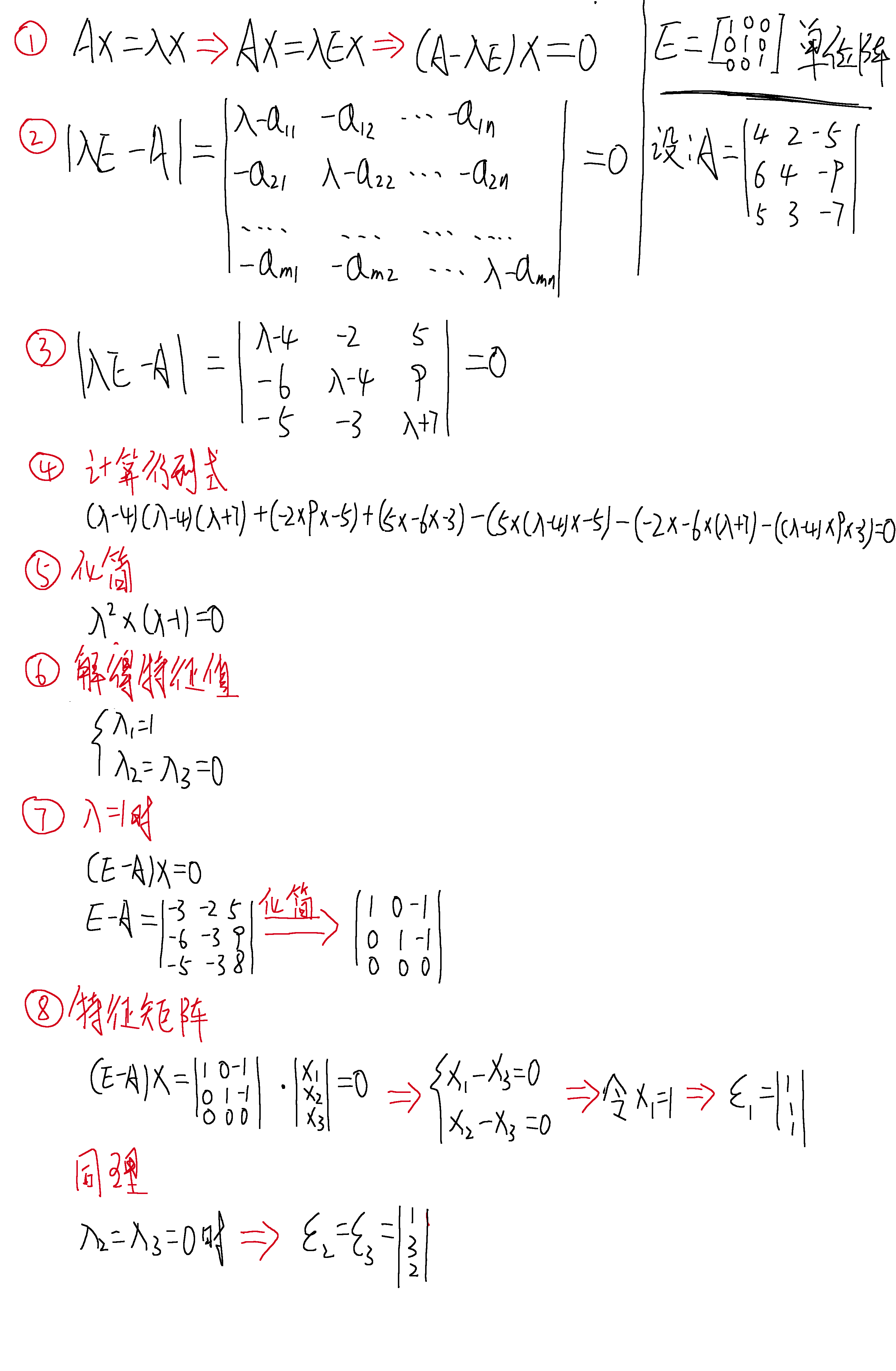展开全文特征矩阵
• 三个向量叉乘的公式 二重积应该都看得懂有手就行 那么三重积应该怎么推导呢？ 首先看标量三重积 标量三重积是三个向量中的一个和另两个向量的...[流氓证法]先bxc 会获得b向量和c向量所在平面的法向量 再叉乘a结
三个向量叉乘的公式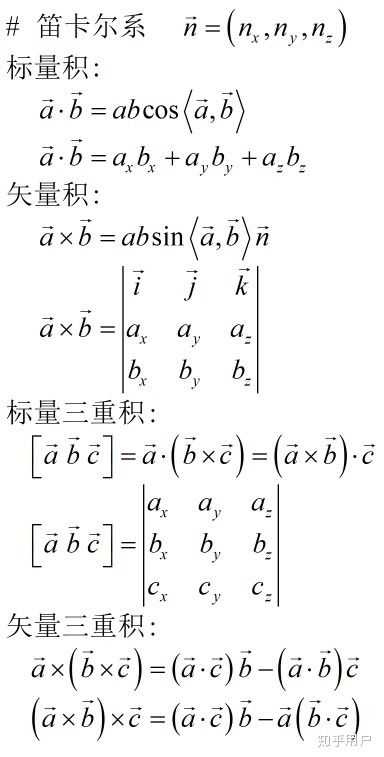二重积应该都看得懂有手就行 那么三重积应该怎么推导呢？

首先看标量三重积
标量三重积是三个向量中的一个和另两个向量的叉积相乘得到点积，其结果是个标量。
设a,b,c为三个向量，则标量三重积定义为 a·(bxc)
证明
a=a1i+a2j+a3k
b=b1i+b2j+b3k
c=c1i+c2j+c3k
用两张图第一张是百度文库的，第二张是我用鼠标写的，因为百度文库少了一步关键的拆解过程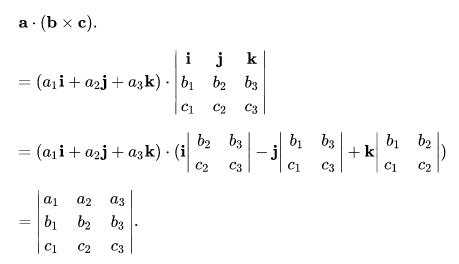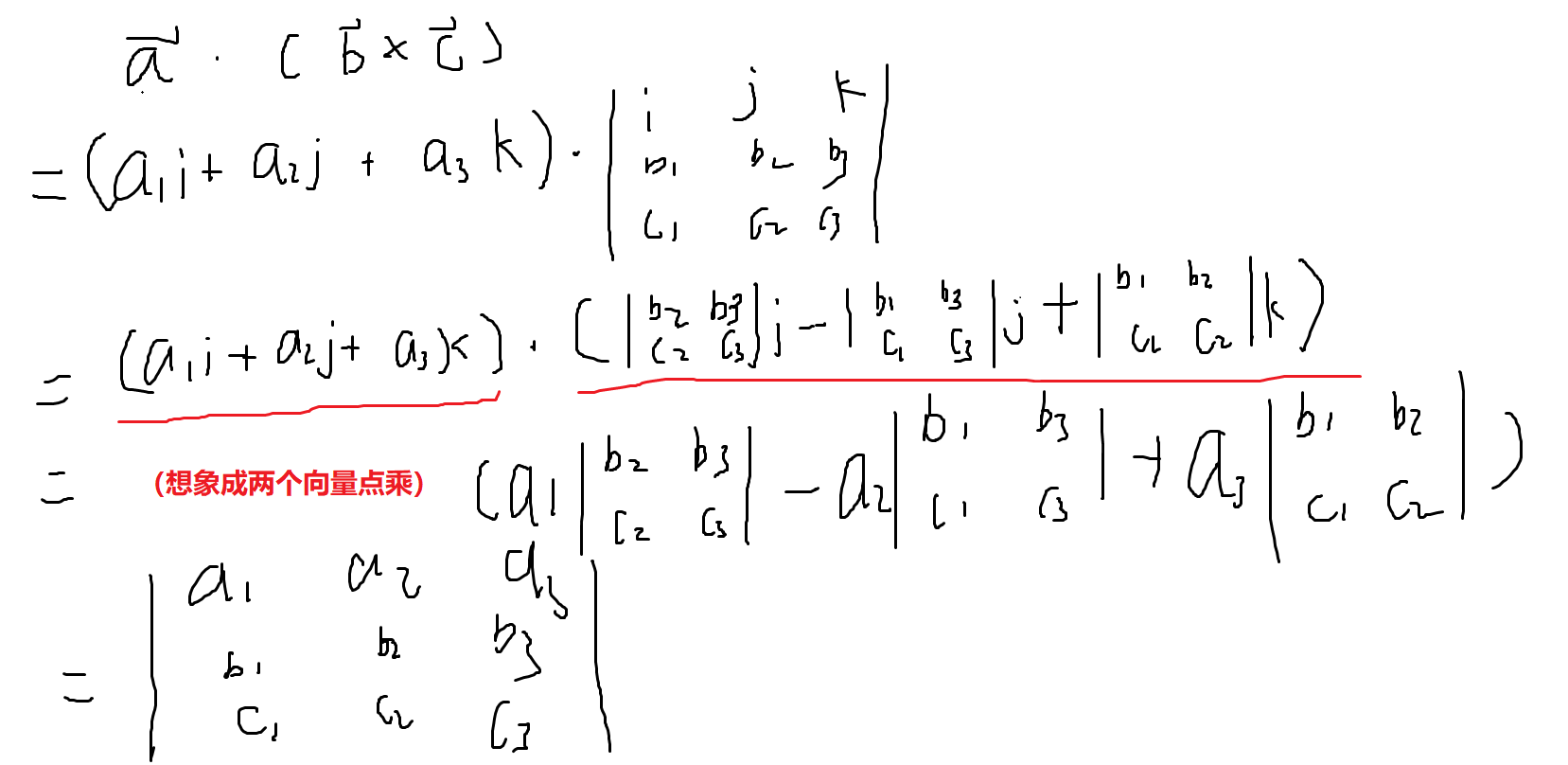然后是矢量三重积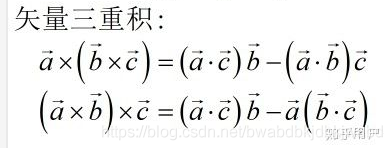[流氓证法]先算bxc 会获得b向量和c向量所在平面的法向量   再叉乘a结果必为b和c所在平面
ax(bxc)=nb+mc
又因为此时的a必垂直（nb+mc）
故 a·（nb+mc）=na·b+ma·c=0
则 n = ac   , m = -ab
故ax(bxc)=(ac) b-(ab)c


展开全文• 在支持向量机（一）和支持向量机（二）两篇文章中，我们分别介绍了线性可分的支持向量机和线性向量机，从中可知，我们最终的目的是要计算得出支持向量对应的拉格朗日乘子α，但是我们并没有说怎么算。这篇文章主要...机器学习
• 支持向量机的理论太多了，特别是KKT条件，不怎么好懂，书上讲得还可以，但是细节还是不够深入，推导过程应该不难，要特别理解对偶这个概念，min max=max min，在取最优值时是相等的，这里面包含了很多数学问题，...
• 写在前面：这篇博文可能更适合于学过线性代数的同学 写作背景：最近开始看花书，第二章就讲到了上面几个概念，主要还是奇异值分解，在看的过程中查找了资料，...（这样读起来好像没啥用，下面会给一个例子来说明怎么算机器学习
• 现在的问题是 如果新来一个特征值 我怎么知道结果 换句话就是说:这个SVC1把结果出来了 ，我怎么利用这个结果去计算新的特征值的结果（不用调包的方法） 调包侠不会使用svc1对象里面的dual_coef ，intercept,...人工智能 机器学习
• top1就是你预测的label取最后概率向量里面最大的那一个作为预测结果，你的预测结果中概率最大的那个类必须是正确类别才预测正确。而top5就是最后概率向量最大的前五名中出现了正确概率即为预测正确。 ...深度学习
• 最近在做李宏毅深度学习2020作业8：Seq2Seq时，非常纠结一点，注意力值出来用在哪儿？ 在作业的BASE版本上加上注意力后，机器翻译的效果并没有提升，因此决定梳理一番。 本文主要关注attention用谁计算，以及计算...自然语言处理 神经网络
• 怎么算都算不出来，直到看到“返回求和...”里的w只有一个i而没有j。好了，那么开始按照题目的要求来做题了。 既然发现了自己的错误，那么就知道了dot-product是干嘛的了，它可以用来求一个矩阵中的一列和一个...SICP
• /* 怎么求夹角；已知两点坐标要求其到原点连线的夹角， 那么我们可以用向量去做...向量的模的乘积怎么算: sqrt（x^2+y^2）; 向量的乘积 x1*x2+y1*y2; */ #include<iostream> #include<cmath> usi...
• 首先解决的问题是怎么算任意多边形的面积，当然是分成小三角形的方式，分别计算再相加。而因为给定的是点的坐标，所以面积表达式应该用向量形式，这里选择的是向量的叉积来计算面积，将每一组坐标储存在数组中，再算...
• I. 二维向量的叉积 设有二维向量和，则 ...把向量1写到第一列，把向量2写到第二列，行列式的结果(行列式就相当于二维向量所围的面积相比原空间缩放的比例) 解释: 说明此正交二维向量叉积的大小就是1...
• 先来说下叉乘怎么算吧，a=（Xa,Ya,Za）,b=(Xb,Yb,Zb),两三维向量的叉乘:|axb|=|a|b]sin<a,b>,axb=(YaZb-ZaYb)i+(ZaXb-ZbXa)j+(XaYb-XbYa)z,而二维向量可以看成三维向量的z为0，则二维向量的叉乘为(XaYb-Xb*Ya)...
• 首先我们想一下如果甲乙都仅沿着两条线段向前跑,那么他们之间的最短和最长距离怎么算? 假设甲的速度向量为v1(速度向量指甲单位时间所走的位移向量),乙的速度向量为v2. 那么可以把甲看成是静止不动的,而让乙一个人以...
• 1.先判断他们是否在一个集合，即父亲是否相同，若父亲相同，用向量算的父亲之间的关系和之前的关心有矛盾没，有矛盾就是有错。 2.如果不在一个集合，就合并他们的父亲，用向量根据根节点的关心求出父亲的关心，然后...
• 思路：先简化版本，如果甲乙都仅沿着两条线段向前跑,那么他们之间的最短和最长距离怎么算? 假设甲的速度向量为v1(速度向量指甲单位时间所走的位移向量),乙的速度向量为v2. 因为运动是相对的，可以把甲看成是静止的，...
• 回顾一下上文的思路，对于线性可分问题，我们可以用超平面将训练样本分开。但是通常超平面有很多个，我们选择距离两类样本点几何间隔最大的...||w||表示向量w的“范数”，最常用的是二阶范数，怎么算呢？就是把向量w...
• 这次我们将学会怎么使用pcl::MomentOfInertiaEstimation...第一次先出点云矩阵的协方差，计算它的特征值和特征向量。然后把特征向量进行归一化处理，并把它组成右手坐标系。每一步都会迭代一次。每一次迭代特征向量
• 对于聚类来说，相似性非常重要，下面我们就来看看相似度怎么算 最经典的就是欧式距离（两点之间的距离，上图中的二维空间公式） 上图中右下角的第一个公式就是Jaccard系数，如果是距离的话第二个公式可以体现 ...
• 一遇到数学题和计算几何题我就要调半天…… 玛雅，我真是太弱了…… 基本思路很简单，先上凸包，然后...后来发现可以用点积来判断，点积的几何意义：向量A在向量B上投影的长度*向量B的长度 然后就很好做了 ...
• 定义你的实例的比较，实现__eq__方法，有一个参数(other),并且返回一个布尔值3.math.hypot接受一个二维向量它的模。4.__iter__方法的实现让这个实例变成一个可迭代对象。也就是说可以for i in 实例了，也可以*...
• 对于线性可分两类数据，支持向量机就是条直线(对于高维数据点就是一个超平面), 两类数据点中的的分割线有无数条，SVM就是这无数条中最完美的一条，怎么样才最完美呢？就是这条线距离两类数据点越远，则当有新的......